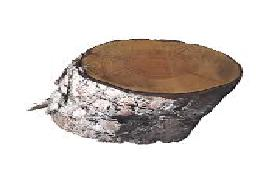# Maintain aspect ratio during grid sample

I am using affine grid and grid sample to rotate an image by 90 degrees. This is the original imageThis is the output of grid sample. The aspect ratio is not maintained.This is the expected output with padding set to zeros.Is there anything else that needs to be considered?

Code to reproduce output:

``````import torch
import numpy as np
import matplotlib.pyplot as plt
import imageio

angle = 90
affine_matrix = np.array(
(
(0, 0, 1)), dtype=np.double
)
rotate_pyorch_2d = torch.from_numpy(affine_matrix[:2, :3]).unsqueeze(0)
grid_2d = torch.nn.functional.affine_grid(rotate_pyorch_2d, img_2d_tensor.shape)
pytorch_resampled_2d = torch.nn.functional.grid_sample(img_2d_tensor, grid_2d, mode="nearest", padding_mode="zeros")
plt.imshow(pytorch_resampled_2d.squeeze().permute(1,2,0).numpy().astype(np.uint8))
``````

I think the result is expected, since your `grid_2d` tensor fills the all pixels of the output tensor, which is why it would stretch the input.
I think you can achieve the last output by setting the `size` in `affine_grid` to the smaller desired size and place the transformed image in the larger empty output.

@ptrblck the angle of rotation can be other than 90 degrees. Also, the white part of the last image is the part of the image itself which is not been displayed in the first two images. In the last image, the whole image is rotated but by maintaining the aspect ratio. Can we set the size to make the rotation work for any degree of rotation?

If I understand the `grid_sample` and `affine_grid` method correctly, you are defining a sampling grid, where each position gives you the pixel location of the input image.
If your grid is thus containing values in all positions, it would just fill these using the values.
Thus, if you don’t want to fill the complete output image (i.e. leave the borders empty to maintain the aspect ratio), you would have to change the grid itself.